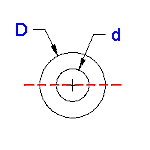# Engineering Section Properties Round Tube at Center

 Variables inputs: Inch (in.) Metric (mm) "D" (O. D. ) "d" (I. D. ) Calculations Moment of Inertia (Units4) = Section Modulus (Units3) = Radius of Gyration (Units) = Extreme Point (Units) = Area (Units2) =